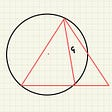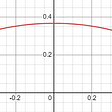# Axis of Symmetry

An imaginary straight line that is dividing a shape into two identical parts is known as the axis of symmetry. One of the identical parts acts as the mirror image of the other one. If the shape is folded along the axis of symmetry, those two identical parts get superimposed. The straight line, also known as the line of symmetry or the mirror line, can be vertical, horizontal, or slanting. In real-life situations, we can observe the axis of symmetry. For example, flowers, riverbanks, leaves, buildings, etc.

A straight line that makes the shape of an object look symmetrical is known as the axis of symmetry. The axis creates the same reflections on either of its sides. The axis can be horizontal, vertical, or lateral. Every shape has a different line of symmetry.

For example, a square has four lines of symmetry, a rectangle has two lines of symmetry, a parallelogram does not have any line of symmetry, and a circle has infinite lines of symmetry. A regular polygon shape with n sides consists of n number of axis of symmetry.

## Axis of Symmetry of a Parabola

The axis of symmetry, which is a straight line, divides the parabola into two symmetrical parts. There are four types of parabolas: horizontal or vertical or facing left, or right. The axis that divides the parabola, determines its form.

• When the axis of symmetry is vertical, the parabola is also vertical.
• If the axis of symmetry is vertical, the parabola is also vertical in shape.
• If the axis of symmetry is horizontal, then it has zero slopes.
• If the axis of symmetry is vertical, its slope is undefined.

## Axis of Symmetry: Equation

The axis of symmetry intersects the parabola at a point which is called the vertex. The vertex is the key point where the equation should be determined. When the parabola opens up or down, then the axis is vertical and its equation is that vertical line that passes through the vertex.

Again, if the parabola opens right or left, the axis of symmetry becomes horizontal and the equation is the horizontal line passing through the vertex.

## Axis of Symmetry: Formula

The formula of the axis of symmetry is applied when the standard form of the equation and the line of symmetry is used. The line that is dividing the object into two halves can be any one of the three different types — horizontal, vertical, or incline line.

The equation of the axis of symmetry is written if the parabola is seen to be in two types, and they are standard form or vertex form.

● Standard Form
The quadratic equation, in standard form, is y=ax2+bx+c, given that a, b and c are the real numbers. And, the formula for the axis of symmetry is x=-b/2a.

● Vertex Form
In vertex form, the quadratic equation is y=a(x-h)2 +k, given that (h, k) is the vertex of the given parabola. Lastly, the formula of the axis of symmetry, in this case, is x=h.

## Math Classes

In today’s generation, especially after the COVID-19 outbreak, online courses have come to the rescue. From kindergarteners to office workers — everyone is now dependent on the Internet. Students conduct their classes online. It becomes more interesting when they have access to websites that offer various articles on learning materials.

However, we generally see students avoiding math when they sit down to study. One reason may be that the foundation of knowledge in mathematics is not strong enough.

Cuemath offers math articles and online math classes that help kids or students understand topics better. Cuemath guides every step so that the students can have fun, and review formulas and calculation examples. To know more about the axis of symmetry, visit Cuemath to book a free session.

--

--

--

## More from The Kashmir Pulse

The Kashmir Pulse is a premier news portal with a reputation for fearless reporting, agenda-setting stories and digital innovation among its global, progressive

Love podcasts or audiobooks? Learn on the go with our new app.

## Difference between PDF and CDF## Curve Sketching## What Is The Area Of The Circle?## Discrete Probability Distribution with Python## The Problem with Monty Hall## The Simplest Math Problem We Still Can’t Solve — Part 3## How Do Casinos Make Money?## What Game Has the Worst Odds at Casinos?## The Kashmir Pulse

The Kashmir Pulse is a premier news portal with a reputation for fearless reporting, agenda-setting stories and digital innovation among its global, progressive

## A Few Technical Notes on Test Functions## Learn Basics of Mathematics Online from Physics Wallah## How to Plot Intersection of Two Cylinders in Maple## Coordinate Geometry Class 9th